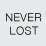# Best Practices: Adding / Calculating many fields in Model Builder?

3905
0
06-04-2012 06:54 AMby
Occasional Contributor III
Hello,

I need to create a tax table that has different schema than the source table.  Basically I am trying to figure out the cleanest way to 1) Add many fields to a table, 2) calculate the new fields based on the values of the old fields, 3) delete the old fields.

One possible solution that I came across is:
1) Include the add field function in my model
2) Right click on "Add Field" then make variable from parameter and add Field Name, Field Type, and Field Length if necessary
3) For variable add the number of variables that I want to populate
4) The add field function will then allow me to add many fields at once.
5) Repeat steps 2-4 for calculate field.

The problem that I have is that in my subsequent "delete field" operation It tries delete each field 50 times (once for each new added field).  The code doesn't fail, but it is inefficient when it attempts to delete a field multiple times when the field only needs to be deleted once.

My question is:

1)  Is this the best practice to add and calculate many fields at once in model builder?

2)  How can I get Model Builder to stop viewing the table as a list.  So that delete field and other operations only try to execute once?

In the Image below when I create variables to add fields and calculate fields subsequent operations still recognize the table as a list, which is not wanted

[ATTACH=CONFIG]14912[/ATTACH]

Thanks
Tags (3)
0 Replies